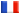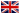# Séminaire de Cryptographie

## Frederik Armknecht### On Fast Algebraic Attacks

An algebraic attack is a method for cryptanalysis which is based on finding and solving a system of nonlinear equations. Recently, algebraic attacks where found helpful in cryptanalysing stream ciphers based on linear feedback shift registers. The efficiency of these attacks greatly depends on the degree of the nonlinear equations.

At Crypto 2003, Courtois proposed fast algebraic attacks. The main idea is to decrease the degree of the equations using a precomputation algorithm. Unfortunately, the correctness of the precomputation algorithm was neither proven, nor was it obvious in all cases.

In the first part of the talk, an introduction to fast algebraic attacks is given. In the second part, the results introduced in the paper "Improving Fast Algebraic Attacks" (FSE 2004) are presented in more detail. This includes the missing proof of correctness and an improvement of the precomputation algorithm. All aspects will be illustrated on the Bluetooth keystream generator E_0.